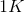# MCAT Physical : Entropy

## Example Questions

### Example Question #1 : Entropy

A scientist prepares an experiment to demonstrate the second law of thermodynamics for a chemistry class. In order to conduct the experiment, the scientist brings the class outside in January and gathers a cup of water and a portable stove.

The temperature outside is –10 degrees Celsius. The scientist asks the students to consider the following when answering his questions:

Gibbs Free Energy Formula:

ΔG = ΔH – TΔS

Liquid-Solid Water Phase Change Reaction:

H2O(l) ⇌ H2O(s) + X

The scientist prepares two scenarios.

Scenario 1:

The scientist buries the cup of water outside in the snow, returns to the classroom with his class for one hour, and the class then checks on the cup. They find that the water has frozen in the cup.

Scenario 2:

The scientist then places the frozen cup of water on the stove and starts the gas. The class finds that the water melts quickly.

After the water melts, the scientist asks the students to consider two hypothetical scenarios as a thought experiment.

Scenario 3:

Once the liquid water at the end of scenario 2 melts completely, the scientist turns off the gas and monitors what happens to the water. Despite being in the cold air, the water never freezes.

Scenario 4:

The scientist takes the frozen water from the end of scenario 1, puts it on the active stove, and the water remains frozen.

After the processes in each of the four scenarios above, what can be said about the entropy of the universe?

It increases in scenarios 1 and 3, only.

It increases in scenarios 1 and 2, only.

It increases in scenarios 2 and 4, only.

It increases in all scenarios.

It increases in scenarios 3 and 4, only.

It increases in scenarios 1 and 2, only.

Explanation:

Scenarios 3 and 4 are presented as thought experiments because, common sense tells us, they will never happen. The fundamental reason they will never happen is because they do not lead to a total increase in entropy of the universe. In order for an event to proceed, this requirement must be satisfied.

### Example Question #2 : Entropy

Which of the following chemical or physical processes involves an increase in entropy?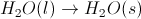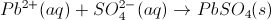Picking up a set of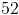playing cards on the floor and stacking them in a deck.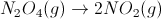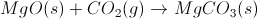Explanation:

Entropy describes the number of configurations a system can have or, alternatively, how much "order" it possesses.

When water freezes, it forms a crystal lattice, which is more ordered than liquid water. Likewise, collecting playing cards and stacking them in a deck involves a transformation from disorder (cards scattered around the floor) to order (a single deck). Chemically, the precipitation of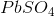from aqueous ions comes from a decrease in entropy, as the free-flowing ions have been combined to form a solid, organized in a lattice. The combination of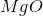and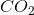to give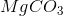also results in a decrease in entropy, since the reactants have one mole of gas, and the products contain zero moles of gas.

The disproportionation of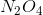to give two moles of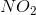results in an increase in entropy, because the number of moles of gas increases in going from reactants to products.

### Example Question #3 : Entropy

Acids and bases can be described in three principal ways. The Arrhenius definition is the most restrictive. It limits acids and bases to species that donate protons and hydroxide ions in solution, respectively. Examples of such acids include HCl and HBr, while KOH and NaOH are examples of bases. When in aqueous solution, these acids proceed to an equilibrium state through a dissociation reaction.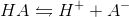All of the bases proceed in a similar fashion.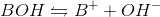The Brønsted-Lowry definition of an acid is a more inclusive approach. All Arrhenius acids and bases are also Brønsted-Lowry acids and bases, but the converse is not true. Brønsted-Lowry acids still reach equilibrium through the same dissociation reaction as Arrhenius acids, but the acid character is defined by different parameters. The Brønsted-Lowry definition considers bases to be hydroxide donors, like the Arrhenius definition, but also includes conjugate bases such as the A- in the above reaction. In the reverse reaction, A- accepts the proton to regenerate HA. The Brønsted-Lowry definition thus defines bases as proton acceptors, and acids as proton donors.

A scientist studying the dissociation of the Arrhenius base sodium hydroxide discovers that the reaction is very exothermic. What is true of the entropy change in the surroundings?

Assume the system refers to the reaction vesselcontaining aqueous sodium hydroxide.

The entropy of the surroundings must remain constant

The entropy of the surroundings may increase or decrease

The entropy of the surroundings is zero

The entropy of the surroundings decreases

The entropy of the surroundings increases

The entropy of the surroundings increases

Explanation:

The entropy change of the surroundings for an exothermic system must be positive. The release of heat by the exothermic reaction drives the increase in disorder of the environment surrounding the reaction vessel.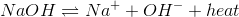As the heat leaves the reaction system, it increases the entropy of the particles in the surroundings.

### Example Question #4 : Entropy

Which of the following chemical processes involves a decrease in entropy?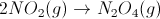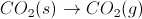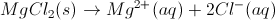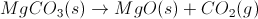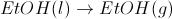Explanation:

Entropy decreases when the number of moles of gas decreases during a reaction. In the case of the correct answer, the number of moles of gas decreases from two to one.

When a substance goes from a solid to a gas (sublimation) or from a liquid to a gas (evaporation), entropy increases. Likewise, when a solid dissolves in water, entropy increases. Entropy (i.e. the number of arrangements a system can have) is much greater in a gas than in a liquid or solid. It is also greater for ions solvated in solution than for an un-dissolved solid.

Note that, in general, the entropy of the universe increases. In order for a process to involve a decrease in entropy of the system, there is likely a consequent increase in entropy of the universe.

### Example Question #5 : Entropy

Which of the following processes involves an increase in entropy?

Sublimation

Freezing

Condensation

More than one of the other options

Crystallization

Sublimation

Explanation:

Entropy is a measure of disorder or randomness. A system with more random motion between molecules has greater entropy. The phases of matter in order of increasing entropy are solid, liquid, then gas. The processes that increase entropy by changing phases will cause a phase transition from lower entropy to higher entropy. These transitions are melting (solid to liquid), vaporization (liquid to gas), and sublimation (solid to gas).

### Example Question #1 : Entropy

Which of the following does not represent an increase in entropy?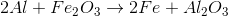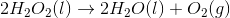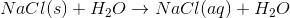A system's temperature increases by